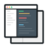# Handwritten Digit Recognition using TensorFlow with Python-1

##### The goal of this tensorflow project is to identify hand-written digits using a trained model using the MNIST dataset. The MNIST dataset contains a large number of hand written digits and corresponding label (correct digit)#### Videos

Each project comes with 2-5 hours of micro-videos explaining the solution.#### Code & Dataset## Customer Love#### Hiren AhirMicrosoft Azure SQL Sever Developer, BI Developer

I'm a Graduate student and came into the job market and found a university degree wasn't sufficient to get a good paying job. I aimed at hottest technology in the market Big Data but the word BigData... Read More#### Shailesh KurdekarSolutions Architect at Capital One

I have worked for more than 15 years in Java and J2EE and have recently developed an interest in Big Data technologies and Machine learning due to a big need at my workspace. I was referred here by a... Read More

## What will you learn

Understanding the problem statement
Understanding One-hot encoded vectors
Converting encoded vector to images using helper function
What is Tensorflow and how does it works
What are Placeholder variables
Understanding a deep learning model and terms associated with it
Softmax activation function
"Gradient Descent Optimizer " and "Cross_entropy" loss function
Defining and Initiating a Tensorflow session
Plotting graphs after each epoch
Plotting graphs for weights after each optimization iteration
Making function for plotting graphs for each Convolution layer
Visualization of Weights and Optimization iteration using Confusion Matrix
Using Seaborn's heatmap function for visualizing Confusion Matrix

## Project Description

Learn computer vision fundamentals with the famous MNIST dataset.

Start here if...
you’re new to computer vision. This tensorflow project is the perfect introduction to techniques like neural networks using a classic dataset including pre-extracted features.MNIST ("Modified National Institute of Standards and Technology") is the de facto “hello world” dataset of computer vision. Since its release in 1999, this classic dataset of handwritten images has served as the basis for benchmarking classification algorithms. As new machine learning techniques emerge, MNIST remains a reliable resource for researchers and learners alike.

The goal of this machine learning project using tensorflow is to correctly identify digits from a dataset of tens of thousands of handwritten images. We encourage you to experiment with different algorithms to learn first-hand what works well and how techniques compare.

Data Introduction:
Each image is 28 pixels in height and 28 pixels in width, for a total of 784 pixels in total. Each pixel has a single pixel-value associated with it, indicating the lightness or darkness of that pixel, with higher numbers meaning darker. This pixel-value is an integer between 0 and 255, inclusive.

The training data set, (train.csv), has 785 columns. The first column, called "label", is the digit that was drawn by the user. The rest of the columns contain the pixel-values of the associated image.

Each pixel column in the training set has a name like pixelx, where x is an integer between 0 and 783, inclusive. To locate this pixel on the image, suppose that we have decomposed x as x = i * 28 + j, where i and j are integers between 0 and 27, inclusive. Then pixelx is located on row i and column j of a 28 x 28 matrix, (indexing by zero).

Practice Skills:
Computer vision fundamentals including simple neural networks.

Acknowledgements:
More details about the MNIST dataset, including algorithms that have been tried on it and their levels of success, can be found at http://yann.lecun.com/exdb/mnist/index.html. The dataset is made available under a Creative Commons Attribution-Share Alike 3.0 license.

## Similar Projects

#### Handwritten Digit Recognition using TensorFlow with Python-2

In this tensorlfow project, our goal is to correctly identify digits from a dataset of tens of thousands of handwritten images.

#### Sequence Classification with LSTM RNN in Python with Keras

In this project, we are going to work on Sequence to Sequence Prediction using IMDB Movie Review Dataset​ using Keras in Python.

#### MNIST Dataset : Digit Recognizer Data Science Project

In this data science project, we are going to work on video recognization data and a robust level of image recognization MNIST data.

4-June-2017
03h 53m